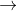# electrochemistry

1. Mn2+ +2H2OMnO2 +4H++2e- E0= -1.23
MnO4- +4H++3e-MnO2+2H2O E0=+1.70

Select correct statement[s]:
a.Mn2+ reacts with MnO4- in acid solution to form MnO2
b.Mn(MnO4)2 is stable in acid soln.
c.MnO2 disproportionates to Mn2+ and MnO4- in acid soln.
d.NOT

2. consider half cell reactions:
H2O2O2+2H++2e- E0=-0.69
H2O2 +2H++2e-2H2O E0=1.77
Fe2+Fe3++e- E0=0.771
FeFe2++2e- E0= 0.44
If H2O2 is mixed with Fe2+, which rxn is more likely
a.Oxidation of Fe2+ to Fe3+
b.Reduction of Fe2+ to Fe
c.Both
d.NOT

3. Cu2++e-Cu+ E0=0.15
Cu2++2e-Cu E0=0.34
predict which exists in aqueous soln
a.Copper(I)Sulphate
b.Copper(II)Sulphate
c.Both
d.NOT

••